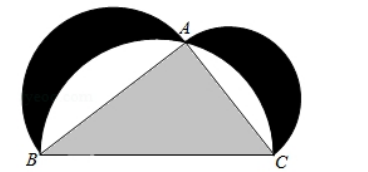A. $\mathrm{p}_{1}=\mathrm{p}_{2}$     B. $\mathrm{p}_{1}=\mathrm{p}_{3}$     C. $\mathrm{p}_{2}=\mathrm{p}_{3}$     D. $\mathrm{p}_{1}=\mathrm{p}_{2}+\mathrm{p}_{3}$